# 二 实现

## 数组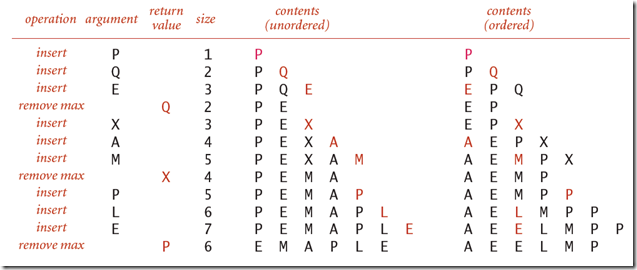· 如果使用无序数组，那么每一次插入的时候，直接在数组末尾插入即可，时间复杂度为O(1)，但是如果要获取最大值，或者最小值返回的话，则需要进行查找，这时时间复杂度为O(n)。

· 如果使用有序数组，那么每一次插入的时候，通过插入排序将元素放到正确的位置，时间复杂度为O(n)，但是如果要获取最大值的话，由于元阿苏已经有序，直接返回数组末尾的 元素即可，所以时间复杂度为O(1).

## 二叉堆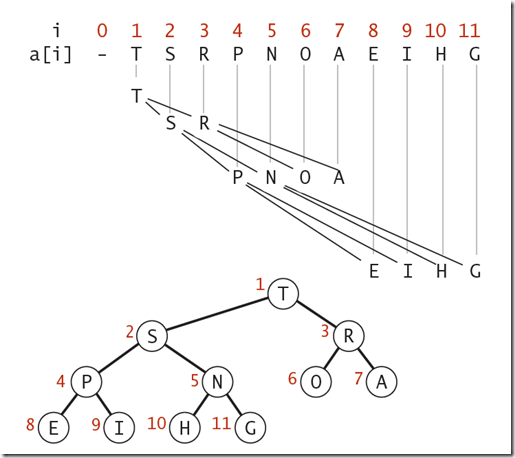· 元素k的父节点所在的位置为[k/2]

· 元素k的子节点所在的位置为2k和2k+1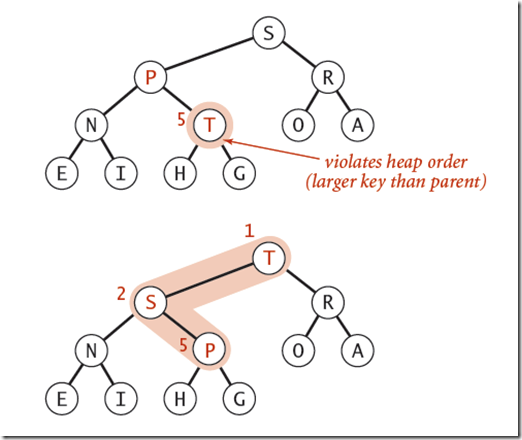``````private static void Swim(int k)
{
//如果元素比其父元素大，则交换
while (k > 1 && pq[k].CompareTo(pq[k / 2]) > 0)
{
Swap(pq, k, k / 2);
k = k / 2;
}
}``````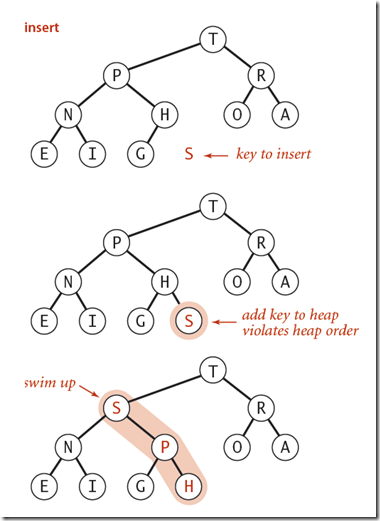``````public static void Insert(T s)
{
//将元素添加到数组末尾
pq[++N] = s;
//然后让该元素从下至上重建堆
Swim(N);
}``````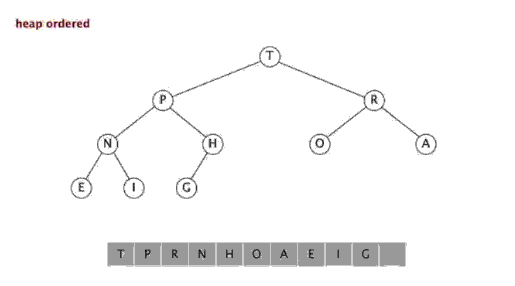``````private static void Sink(int k)
{
while (2 * k < N)
{
int j = 2 * k;
//去左右子节点中，稍大的那个元素做比较
if (pq[j].CompareTo(pq[j + 1]) < 0) j++;
//如果父节点比这个较大的元素还大，表示满足要求，退出
if (pq[k].CompareTo(pq[j]) > 0) break;
//否则，与子节点进行交换
Swap(pq, k, j);
k = j;
}
}``````

\1. 移除二叉堆根节点元素，并返回

\2. 将数组中最后一个元素放到根节点位置

\3. 然后对新的根节点元素进行Sink操作，直到满足二叉堆要求。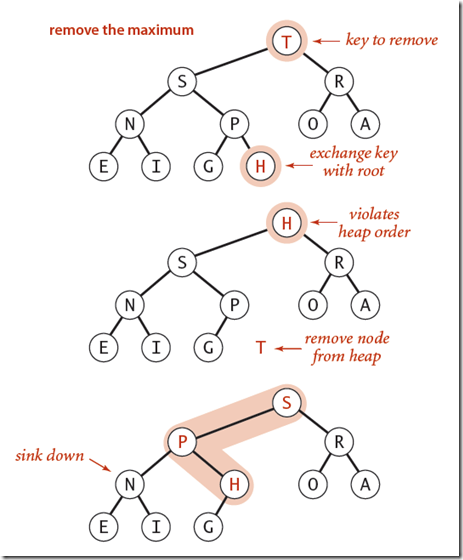``````public static T DelMax()
{
//根元素从1开始，0不存放值
T max = pq;
//将最后一个元素和根节点元素进行交换
Swap(pq, 1, N--);
//对根节点从上至下重新建堆
Sink(1);
//将最后一个元素置为空
pq[N + 1] = default(T);
return max;
}``````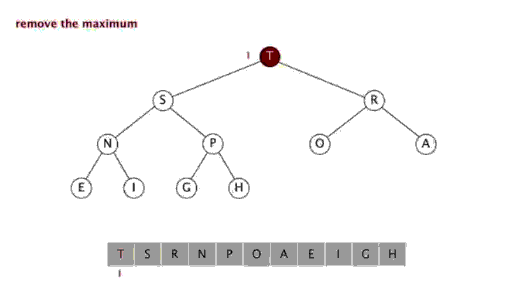# 三 堆排序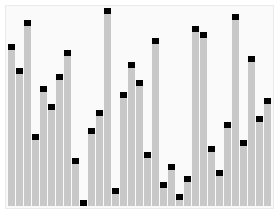## 概念

\1. 使用序列的所有元素，创建一个最大堆。

\2. 然后重复删除最大元素。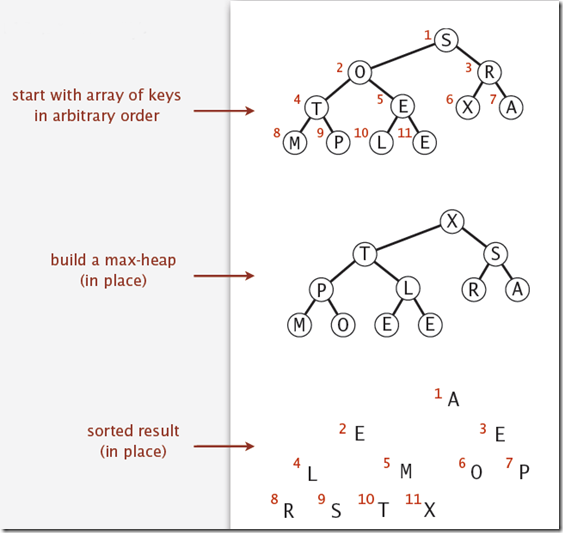``````for (int k = N / 2; k >= 1; k--)
{
Sink(pq, k, N);
}````````````while (N > 1)
{
Swap(pq, 1, N--);
Sink(pq, 1, N);
}``````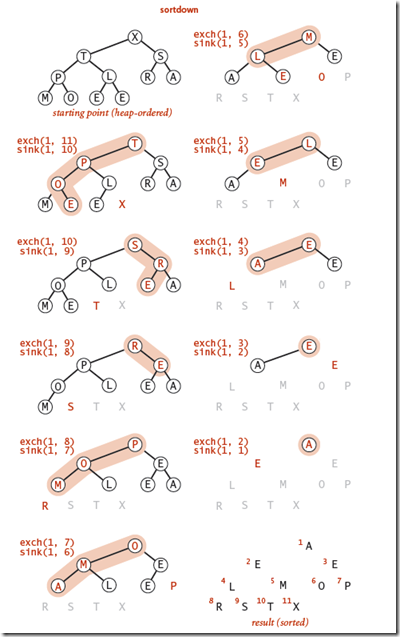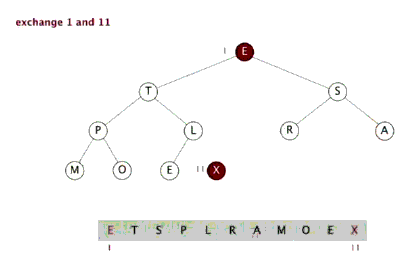## 分析

\1. 在构建最大堆的时候，最多需要2N次比较和交换

\2. 堆排序最多需要2NlgN次比较和交换操作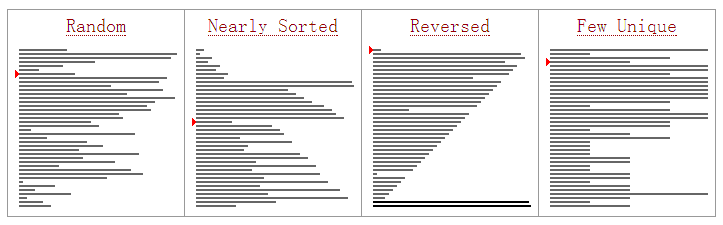\1. 其内部循环要比快速排序要长。

\2. 并且其操作在N和N/2之间进行比较和交换，当数组长度比较大的时候，对CPU缓存利用效率比较低。

\3. 非稳定性排序。

# 四 排序算法的小结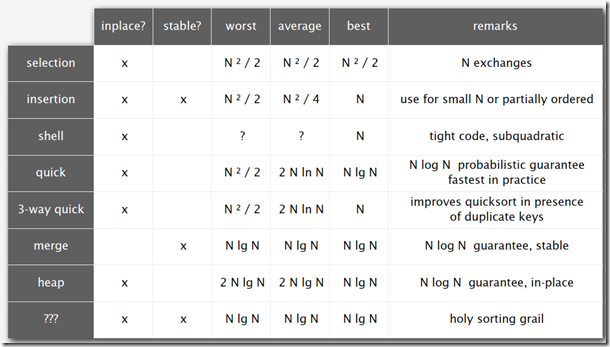# 五 结语

Do you have any suggestions for improvement?